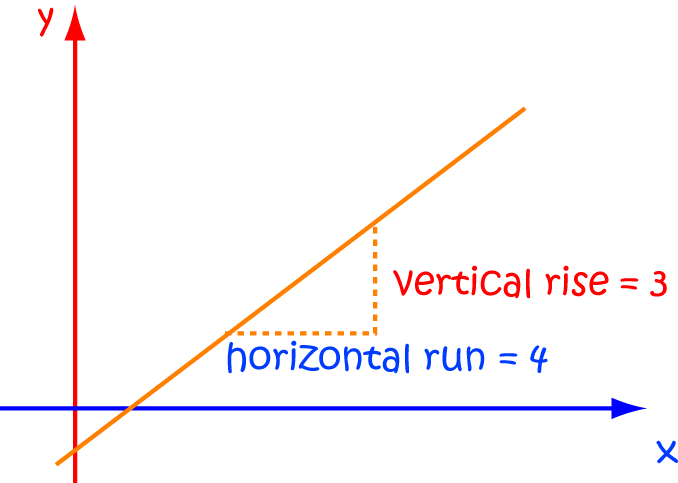# Definition of Horizontal RunThe Horizontal Run of a straight line is the horizontal (left or right) distance it covers for a given vertical (upward or downward) distance.

The gradient (or slope) of a line is given by $\dfrac{\text{Vertical Rise}}{\text{Horizontal Run}}$.

In some countries, "Horizontal Run" is shortened to "run".

### Description

The aim of this dictionary is to provide definitions to common mathematical terms. Students learn a new math skill every week at school, sometimes just before they start a new skill, if they want to look at what a specific term means, this is where this dictionary will become handy and a go-to guide for a student.

### Audience

Year 1 to Year 12 students

### Learning Objectives

Learn common math terms starting with letter H

Author: Subject Coach
You must be logged in as Student to ask a Question.